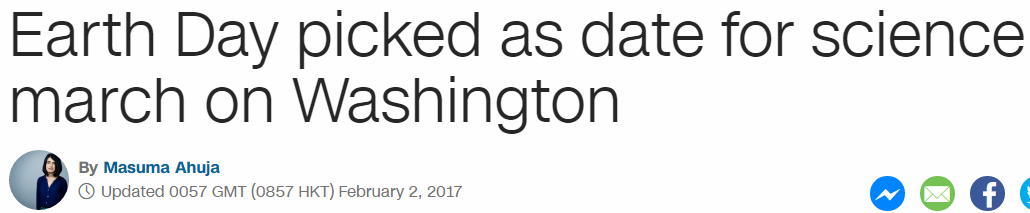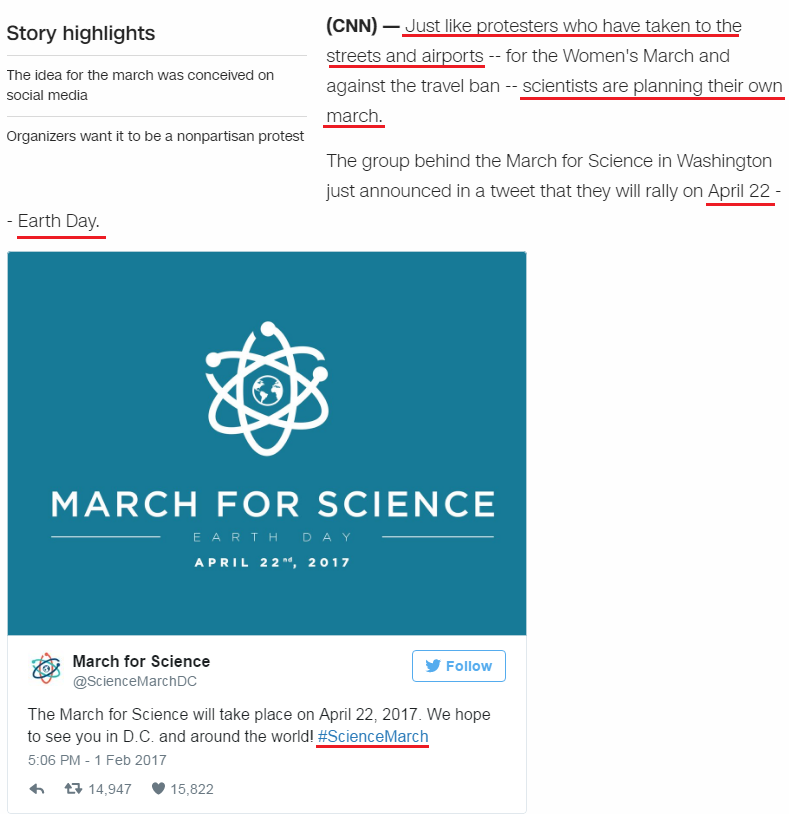## Cipher## Feb 2, 2017

### CNN - Earth Day picked as date for science march on Washington

CNN's post - http://edition.cnn.com/2017/02/01/politics/science-march-earth-day-trnd/index.htmlThe news comes on 33rd day of the year
February Second = 6+5+2+9+3+1+9+7 + 1+5+3+6+5+4 = 66 (Reduced)
February Second = 6+5+2+18+21+1+18+25 + 19+5+3+15+14+4 = 156 (Ordinal)
Thirty Three = 2+8+9+9+2+7 + 2+8+9+5+5 = 66 (Reduced)
Thirty Three = 20+8+9+18+20+25 + 20+8+18+5+5 = 156 (Ordinal)Title of their post has the same gematria as the first line and you can see why it is written so weirdly
Just like protesters who have taken to the streets and airports = 227 (Reduced)
Earth Day picked as date for science march on Washington = 227 (S exception on Washington)
'Twenty-two divided by seven' = 314, 'Seven hundred seventy seven' = 314

The post comes on 2/2 (22nd prime number is 79) from today to the march on 4/22 is 79 days -2 months and 20 days
April Twenty Second = 1+7+9+9+3 + 2+5+5+5+2+7 + 1+5+3+6+5+4 = 79 (Reduced)
April 22nd is 112th day of the year (11x2) - and it is also 11 weeks and 2 days from nowIt's unbelieveable that 4/22 leaves 253 days (22nd triangular number)

But there's more things tied into the gematria of this date of course
April Twenty Second = 1+16+18+9+12 + 20+23+5+14+20+25 + 19+5+3+15+14+4 = 223 (Ordinal)
Last part the of first paragraph also matches this gematria
scientists are planning their own march. = 100+3+9+5+50+200+9+100+200+100 + 1+90+5 + 70+30+1+50+50+9+50+7 + 200+8+5+9+90 + 60+500+50 + 40+1+90+3+8 = 2203 (English)
There's interesting reverse ordinal gematria for the #ScienceMarch from the Tweet
#ScienceMarch = 8+24+18+22+13+24+22+14+26+9+24+19 = 223 (Reverse Ordinal)
223 is number of the synagogue of Satan and 322 is number of Skull and Bones (223+232+322 = 777)

April Twenty Second = 1+70+90+9+30 + 200+500+5+50+200+700 + 100+5+3+60+50+4 = 2077 (English)
#ScienceMarch = 90+3+9+5+40+3+5+30+1+80+3+8 = 277 (Jewish)

The tweet says 5:06 PM which is interesting considering reduced gematria of ScienceMarch
#ScienceMarch = 1+3+9+5+5+3+5+4+1+9+3+8 = 56 (Reduced)
April = 1+16+18+9+12 = 56 (Ordinal)

This is the rest of their post